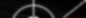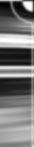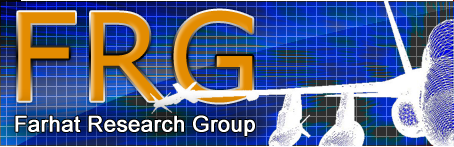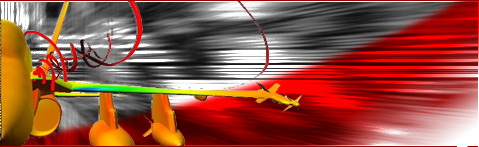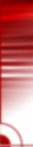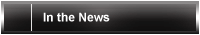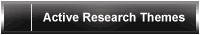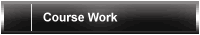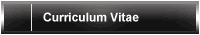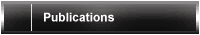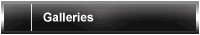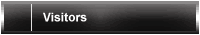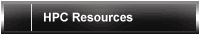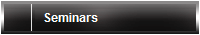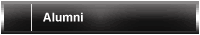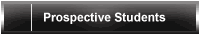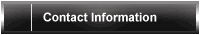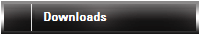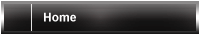Seminars

# Farhat Research Group Seminars

At these meetings, a Farhat Research Group team member will give a 2045 minute talk on their current research. The goal of these seminars is to promote feedback from the whole lab and improve collaboration.

Email me at rtezaur@stanford.edu if you would like to present.

## Upcoming Talks

 Date & time Room Speaker 12/15/2015 3:00-4:00pm Durand 026 Raunak Borker

## Upcoming Talk Abstracts

• 12.15.15 | Raunak Borker

 S. Brogniez, C. Farhat, E. Hachem, A high-order discontinuous Galerkin method with Lagrange multipliers for advectionâdiffusion problems, Computer Methods in Applied Mechanics and Engineering, 264, 49-66, 2013.

## Past Talks

• 12.8.15 | Matthew Zahr

High-order methods for optimization and control of conservation laws on deforming domains

PDE-constrained optimization has become an essential tool in the design of steady-state engineering systems, but is less commonly used for unsteady problems due to the large cost associated with repeatedly solving the underlying PDE. However, there is a large-class of problems that are inherently unsteady, such as flow past airfoils at a high angle of attack, flow through wind farms, and flapping flight, that would benefit from numerical optimization. In this work, a globally high-order numerical scheme is developed for the discretization of conservation laws on deforming domains, and the corresponding fully-discrete adjoint equations are derived. The numerical scheme is used to compute relevant quantities of interest of the conservation law, and their gradients, for the use in gradient-based, nonlinear optimization. I will demonstrate the framework on a range of relevant problems, including the design of energetically optimal flapping wing motions in viscous, compressible flow, subject to various constraints such as prescribed thrust forces. I will also show examples of shape optimization and time-morphed geometries, and how to incorporate time-periodic constraints.

• 8.31.15 | Johanna Ehlers

Optimization of the double-T root of a steam turbine blade through camber of contact surface with ANSYS Workbench

• 8.7.15 | Isaac Harari

Embedded Methods for Plate Bending by Nitsche's Approach

An efficient procedure for embedding kinematic boundary conditions in plate bending is based on a stabilized variational formulation, obtained by Nitsche's approach for enforcing boundary constraints. Work-conjugate pairs weakly enforce kinematic boundary conditions.

For thin plate problems, the absence of kinematic admissibility constraints allows the use of non-conforming meshes with non-interpolatory approximations, thereby providing added flexibility in addressing higher continuity requirements. The use of a scaling factor leads to a formulation with a single stabilization parameter. The enforcement of tangential derivatives of deflections obviates the need for pointwise enforcement of corner values in the presence of corners. The single stabilization parameter is determined from a local generalized eigenvalue problem, guaranteeing coercivity of the discrete bilinear form. The accuracy of the approach is verified by representative computations with bicubic B-splines, providing guidance to the determination of the scaling and exhibiting optimal rates of convergence and robust performance with respect to values of the stabilization parameter.

For shear-deformable plates, the absence of kinematic admissibility constraints facilitates the use of approaches that are insensitive to shear locking. Here, the scaling factor is taken as the plate thickness. This leads to a formulation with a single stabilization parameter, which is determined from a local generalized eigenvalue problem, guaranteeing coercivity of the discrete bilinear form.

• 8.7.15 | Tommaso Tamarozzi

On the efficient simulation of flexible gears using model order reduction

The performance of a mechanical transmission is largely governed by the dynamic behavior of its main constituents, being the gears and bearings. These complex rotating components transmit power through mechanical contact interactions that are accompanied by elastic deformations and high dynamic stresses. Accurate prediction of these quantities under a variety of operating conditions is indispensable for reliable and cost-effective gearbox design, both in automotive, aerospace and wind-energy industries. Standard lumped-parameters models or rigid multibody approaches do not provide a sufficient level of details to study the dynamic effects induced by e.g. gear design modifications (micro-geometry) or to analyze local stress concentrations. More advanced numerical tools are available such as flexible multibody or non-linear FE and allow to model complex contact interactions including all the relevant dynamic effects. Unfortunately the level of mesh refinement needed for an accurate analysis causes these simulations to be computationally expensive with time scales of several weeks to perform a single full rotation of a gear pair.

After revising the typical workflow of computer programs dealing with contact problems and categorizing the most used approaches and their advantages and disadvantages, this seminar introduces both from a theoretical and applied point of view a novel efficient simulation method for dynamic analysis of transmissions. This method adopts a flexible multibody paradigm but incorporates several advanced features that allows to run simulations up to two orders of magnitudes faster as compared to non-linear FE with the same level of accuracy. A non-linear parametric model order reduction technique is used to develop a simulation strategy that is quasi mesh- independent allowing the usage of very fine FE meshes. Issues like memory and CPU time will also be briefly treated. The method will be used to demonstrate the importance of capturing continuous and discontinuous non-linear phenomena and underline the importance of dynamic effects for highly transient scenarios such as voltage dips in wind turbines. Several academic and industrially relevant examples coming from the wind energy industry will be used as show cases.

• 6.9.15 | Kevin Carlberg

Integrating reduced-order models with uncertainty quantification: modeling and controlling error

Many tasks in uncertainty quantification require hundreds or thousands of `forward' model simulations. In Bayesian inference for data assimilation, for example, each sample from the posterior distribution requires (at least) one forward simulation. Employing high-fidelity, large scale computational models for such tasks is infeasible, as a single simulation can consume days or weeks on a supercomputer.

To make such problems tractable, we 1) replace the large-scale model with a low-dimensional projection-based reduced-order model (ROM), 2) rigorously account for the additional (epistemic) uncertainty introduced by the ROM, and 3) control this uncertainty by adaptively refining the ROM as needed.

Two newly developed methods enable this strategy. For step 2 above, we propose the ROM error surrogate (ROMES) method , which employs stochastic processes to construct accurate statistical models of the ROM error. For step 3, we propose an adaptive h-refinement approach  that borrows many concepts from adaptive mesh refinement.

• 3.20.15 | Peng Chen

Sparse grid, reduced basis Bayesian inversion for nonlinear problems

We present a computational reduction framework for efficient and accurate solution of Bayesian inverse problems on high- or infinite-dimensional parameter spaces that commonly face the curse of dimensionality and large-scale computation. For the approximation of high or infinite dimensional integration, we take advantage of sparsity in the parametric solution maps in novel dimension-adaptive sparse grid interpolation and quadrature algorithms. For large scale problems, we also exploit intrinsic sparsity in the solution map and the high-fidelity approximation and propose a novel, goal-oriented reduced basis method. To resolve the nonaffinity and nonlinearity of the underlying problems, we propose nodal empirical interpolation approximation and demonstrate its convergence rate.

• 3.10.15 | Matthew Zahr

A Nonlinear Trust Region Framework for PDE-Constrained Optimization Using Progressively-Constructed Reduced-Order Models

The large computational cost associated with high-fidelity simulations has limited their use in many-query scenarios (optimization and UQ). A nonlinear trust-region framework using Reduced-Order Models (ROMs) is introduced as a means to accelerate PDE-constrained optimization. A progressive approach is employed to construct a ROM during the optimization procedure. On problems from aerodynamic shape optimization, the framework reduces the number of queries to the high-dimensional model by a factor of 4 with no loss in accuracy. Preliminary extension to topology optimization will also be discussed.

• 1.21.15 | Frank Naets

Reduced order mechanical models for virtual measurements

Virtual measurement channels allow to extend existing measurements on mechanical systems with vastly more information through the use of model information. In mechanical applications there are many quantities which are difficult to measure directly because the specific sensors might be costly or intrusive to the system (e.g. force measurements) and for which virtual measurements can provide a practical solution. At the KU Leuven - PMA group efforts are being undertaken to build virtual sensors for advanced mechanical applications where measurements on a system level can be exploited to extract localized information through the use of advanced models. However, due to the high frequencies and nonlinearities present in these models, computational loads are typically high. In order to reach online, and even real-time, performance model reduction is required.

The mechanical problems considered here typically exhibit some form of geometric nonlinearity and have several constraints, such that they are described by a set of nonlinear differential algebraic equations, which are expensive to solve. In order to obtain good performance for virtual sensing purposes, the reduction algorithm should create a set of much smaller (nonlinear) ordinary differential equations. For this purpose the Global Modal Parameterization (GMP) approach has been developed at PMA. This interpolation based approach performs a system level nonlinear model reduction for flexible multibody systems and can provide real-time performance. Due to the ODE structure of the resulting equations this method is perfectly suited for these virtual sensing purposes. However, the GMP approach also has some important limitations of which a brief overview is presented as well.

• 12.9.14 | Kyle Washabaugh

On weakly enforced boundary conditions for discrete nonlinear aerodynamic reduced-order models

Difficulties associated with weakly enforced boundary conditions for parameter-dependent reduced-order models (ROMs) are discussed. A preliminary method for addressing these difficulties is proposed in the context of minimum-residual ROMs for nonlinear Computational Fluid Dynamics (CFD) applications. In the proposed method, the minimum-residual least-squares problem is iteratively weighted to ensure that all weakly enforced boundary conditions are respected. Difficulties with weakly enforced boundary conditions are demonstrated for reduced-order simulations of airfoils with variable shape and variable free-stream angle of attack. For these applications, the proposed method is shown to deliver improved results at predictive operating points.

• 12.2.14 | Raunak Borker

A high-order discontinuous Galerkin method with Lagrange multipliers for advection-diffusion Problems

A high-order Discontinuous Galerkin method with Lagrange Multipliers (DGLM) is presented for the solution of steady and unsteady advection-diffusion problems in the high Peclet number regime. Like the Discontinuous Enrichment Method (DEM), it chooses the basis functions among the free-space solutions of the homogeneous form of the governing differential equation, and relies on Lagrange multipliers for enforcing a weak continuity of the approximated solution across the element interface boundaries. This higher-order DG framework delivers both the expected better accuracy for fixed spatial and temporal resolutions, and a significantly better computational efficiency for a fixed level of accuracy. This is because it operates directly on the second-order form of the governing equation, does not require stabilization, and approximates the solution locally using free-space solution of the homogeneous form of the governing equations rather than polynomials which reduces the constant of its convergence rate. This talk will discuss the theoretical and computational aspects of the method, and results comparing DGLM to a continuous polynomial FEM method will be shown.

• 11.19.14 | Eli Turkel

Time reversal for identification of source and obstacles

Time reversal is based on advancing the solution of the relevant wave problem "backward in time". One important use of numerical time-reversal is that of refocusing, where a reverse run is performed to recover the location of a source applied at an initial time based on measurements at a later time. Usually, only partial, noisy, information is available, at certain measurement locations, on the field values that serve as data for the reverse run. The question concerning the amount and characterization of the available data needed for a successful refocusing is discused for various wave-like equations. A simple procedure is proposed which exploits multiple measurement times, and is shown to be very beneficial for refocusing. A tradeoff between availability of spatial and temporal information is discussed. The effect of measurement noise is studied, and the technique is shown to be quite robust, sometimes even in the presence of very high noise levels. The use of the technique as a basis for scatterer identification is also discussed. A numerical study of these effects is presented,

• 11.4.14 | Matthew Zahr

Fast algorithms for consistent reduced-order bases via proper orthogonal decomposition and low-rank SVD updates: application to local model reduction

An investigation into the offset used in the affine subspace approximation in Proper Orthogonal Decomposition (POD)-based model reduction is performed. A notion of consistency is defined among the affine offset, Reduced-Order Basis (ROB), and set of snapshots from which the ROB is constructed. Four numerical examples are employed to demonstrate the importance of consistency, ranging from illustrative contrived examples to large-scale CFD problems. A bottleneck in the offline-online decomposition of model reduction occurs when the initial condition of a simulation is an online quantity, particularly if consistent reduced-order bases are desired.

Two algorithms for efficiently enforcing consistent reduced-order bases are introduced. The first constructs static affine subspaces offline and leverages precomputations to efficiently perform all required projections. The second approach uses fast, low-rank SVD modifications to efficiently use the online initial condition as the affine offset and enforce consistency. The potential of the two algorithms to efficiently enforce consistency, and generate more accurate reduced-order models for a given basis size than inconsistent counterparts, will be demonstrated on the four numerical examples.

• 10.28.14 | Todd Chapman

ECSW with accelerated and scalable generation of reduced meshes

Hyper-reduction of nonlinear structural ROMs is an active research area with exciting applications in industrial scale problems. Currently, there are two primary approaches to nonlinear hyper-reduction. The first approach is to approximate the nonlinear quantity and project onto the appropriate reduced order subspace. The alternative approach is to project the nonlinear quantity into the reduced order subspace and then introduce the approximation. Energy conserving sampling and weighting (ECSW) takes the latter approach, constructing low dimensional nonlinear functions using a small subset of the original finite element mesh at a fraction of the computational cost of the unapproximated system.

Determining the optimal subset of elements required for an efficient and accurate hyper-reduced model is challenging. It ultimately results in a L0 minimization problem that is known to be NP-hard. Fortunately, the compressed sensing community has developed a wide variety of algorithms which achieve the same result as L0 minimization by instead minimizing the L1 norm. A quantitative comparison has been made between several well know L1-minimization algorithms to determine their suitability for computing reduced meshes for the ECSW method.

Furthermore, in the compressed sensing community, little effort is expended on developing algorithms which are easily parallelizable for distributed memory architectures. This is due to the fact that the systems of equations that result in signal/image processing have fast implicit representations and do not explicit storage. A method of solving the least squares problem, that results at each iteration of nearly every L1 minimization algorithm, via conjugate gradients is given and demonstrated to be an effective way of parallelizing the computation of a reduced mesh.

• 10.21.14 | Arthur Paul-Dubois-Taine

Parameterization framework for aeroelastic design optimization of wing structures

Recent advances in manufacturing technologies such as 3D printing or Electron Beam Melting have given airplane makers much greater design freedom, paving the way to the manufacturing of more complex and more efficient internal aircraft structures. Such technologies are also being used to manufacture insect-like wings for flapping Unmanned Air Vehicles, a field that has gained a lot of interest in the past few years.

This project aims at developing a general optimization framework for complex wing structures, both for classical fixed wing aircraft and flapping UAVs. The framework relies on an automated structural model generation tool associated with a simple but powerful wing structure parameterization. The Aero suite of code is then used to compute aeroelastic solutions affecting the desired objective function. This talk will present details about the design and optimization framework and present preliminary results for fixed wing aircraft optimization.

• 10.14.14 | David Amsallem

Design optimization using hyper-reduced-order models

Solving large-scale PDE-constrained optimization problems presents computational challenges due to the large dimensional set of underlying equations that have to be handled by the optimizer. Recently, projection-based nonlinear reduced-order models have been proposed to be used in place of high-dimensional models in a design optimization procedure. The dimensionality of the solution space is reduced using a reduced-order basis constructed by Proper Orthogonal Decomposition. In the case of nonlinear equations, however, this is not sufficient to ensure that the cost associated with the optimization procedure does not scale with the high dimension. To achieve that goal, an additional reduction step, hyper-reduction is applied. Then, solving the resulting reduced set of equations only requires a reduced dimensional domain and large speedups can be achieved. In the case of design optimization, it is shown in this paper that an additional approximation of the objective function is required. This is achieved by the construction of a surrogate objective using radial basis functions. The proposed method is illustrated with two applications: the shape optimization of a simplified nozzle inlet model and the design optimization of a chemical reaction.

• 10.7.14 | Alex Main

Implicit and higher order discretization methods for compressible multi-phase and fluid-structure interaction problems

This thesis examines the design and implementation of embedded boundary methods for problems involving multiphysics, specifically those involving fluid-fluid and fluid-structure interactions, with an emphasis on those problems involving complex, highly nonlinear phenomena. This thesis makes a variety of contributions to embedded boundary methods. First, an implicit time stepping scheme for embedded boundary methods is designed and implemented, showing speedups of 40x over the comparable explicit counterpart for problems. This framework is validated on problems involving hydrostatic implosions. Then, a second order accurate embedded boundary method is introduced for fluid-fluid and fluid-structure interaction. Finally the accuracy and stability of these embedded boundary methods are examined, by introducing tools to quantify the stability of embedded boundary methods, and techniques are introduced to stabilize embedded boundary methods as necessary.

• 9.19.14 | Daniel Neumann

An iterative approach for CFD-based aeroelastic computations in the frequency domain and its application to design optimization

The objective of the project is the efficient analysis of flutter properties for structures under certain (flight) conditions. The solution of the underlying Lagrange and Euler equations in the frequency domain allows us to directly compute and output the aeroelastic frequencies and damping ratios for the structural modes considered. The outcomes are compared to computational results in the time domain and experimental results based on wind tunnel tests. An application for the implemented approach is shown by applying it to the design optimization procedure of the ARW2 wing.

• 3.12.14 | Guglielmo Scovazzi

Piecewise linear finite elements; arbitrary Lagrangian-Eulerian methods; variational multi scale methods; fluid/structure interaction

A new tetrahedral finite element for transient dynamic computations of fluids and solids is presented. It utilizes the simplest possible finite element interpolations: Piece-wise linear continuous functions are used for displacements and pressures (P1/P1), while the deviatoric part of the stress tensor (if present, as in the case of solids) is evaluated with simple single-point quadrature formulas. The variational multiscale stabilization eliminates the pressure checkerboard instabilities affecting the numerical solution in the case of the Darcy-type operator related to compressible fluids computations, or the Stokes-type operator related to solid dynamics computations. The formulation is extended to strong shock computations in fluids and to elastic-plastic flow in solids. Extensive tests of shock flows in fluids, and of linear elasticity and nonlinear (finite) elastoplasticity (compressible as well as nearly incompressible) will be presented. Because of its simplicity, the proposed FEM method could favorably impact complex geometry, fluid/structure interaction, and embedded discontinuity computations. Time permitting, a number of preliminary results on fluid-structure interaction problems, and transient computations of viscoelastic solids will also be presented.

• 2.26.14 | Mirko Myllykoski

Cyclic Reduction Type Poisson and Helmholtz Solvers on a GPU

The topic of the presentation are the block cyclic reduction type linear system solvers and how they can be applied to the Poisson and Helmholtz equations on a Graphics Processing Unit. The presented implementations are based on a method called radix-q PSCR. A total of three implementations are presented: a simplified radix-2 method, a simplified radix-4 method and a generalized radix-4 method. The presentation will include a short overview to GPU computing using OpenCL. The main matter of the presentation are the GPU implementations and the obtained numerical results.

• 2.5.14 | Patrick Lea

XFEM Primer to New Hybrid Method

This talk covers the extended finite element method (XFEM) starting with the basic building blocks. XFEM is a method of of modelling discontinuities within a finite element mesh. It has been used for both strong (fracture and dislocations) and weak discontinuities (material interfaces, grain boundaries, etc). Two new approaches will be discussed. The first approach is in the determination of the direction of crack propagation. The second approach uses a new concept of looking at propagation and combines it with a method to model branches and junctions. Results from academic problems as well as more real world situations will be presented.

• 12.11.13 | Matthew Zahr

Rapid nonlinear topology optimization using precomputed reduced-order models

Topology optimization of structural systems is an important tool in engineering design to find the most efficient design of a structure for its intended use. The high-dimensional nature of practical computational mechanics (CM) simulations make structural topology optimization a very large-scale nonlinear program with non-convex constraints defined by the discretized, nonlinear continuum equations. It is well-known that such large-scale, non-convex nonlinear programs are difficult and computationally prohibitive to solve. Despite the high-dimensional nature of many CM models, the solution trajectory is typically confined to a low-dimensional affine subspace. This is the inherent assumption in Model Order Reduction (MOR), whereby a Reduced Order Model (ROM) is constructed with many fewer degrees of freedom than the original High-Dimensional Model (HDM), without significant loss of fidelity. The contribution of this work is a framework for using a reduced-order model in a topology optimiza- tion problem, with an emphasis on nonlinear mechanical behavior. To speedup the topology optimization problem, all quantities scaling with the HDM must be reduced, namely, the state vector (generalized dis- placements), governing equations, and optimization variable (usually defined over the elements of the mesh). In the proposed approach, Proper Orthogonal Decomposition (POD) and the method of snapshots are used to construct a reduced basis, which defines the reduction of the state variable. We assume that all nonlinear terms (internal force vector and tangent stiffness matrices) are polynomial in the state vector (generalized displacements). This polynomial assumption enables all terms scaling with the HDM to be precomputed and defines the reduction of the governing equations. Finally, the optimization variables are reduced using POD with snapshots defined a-priori using manufacturability constraints to drive the snapshot selection. The reduction of these three quantities collectively define the reduced topology optimization problem. This presentation will give a brief introduction to reduced-order models and topology optimization, cover the formulation of the proposed method as well as the underlying theory, and demonstrate the effectiveness of the method in rapidly designing a 3D wing box using loads that induce large deformations (over 500Ã speedup with error on the order of 1%).

• 11.19.13 | Dalei Wang

Fully implicit and semi-implicit hybrid discontinuous space-time Galerkin methods for acoustic wave propagation

Medium frequency regime and multi-scale wave propagation problems have been a subject of active research in computational acoustics recently. New techniques have attempted to overcome the limitations of existing discretization methods that tend to suffer from dispersion. One such technique, the discontinuous enrichment method (DEM), was developed for frequency domain problems such as the Helmholtz equation. DEM incorporates features of the governing partial differential equation in the approximation, in particular, the solutions of the homogeneous form of the equation. In this dissertation, based on this concept and on the conventional space-time finite element method, a hybrid discontinuous Galerkin method (DGM) for the numerical solution of transient problems governed by the wave equation in two and three spatial dimensions is described. This hybrid DGM extends concepts of the existing DEM into time domain for problems that are better suited for analysis in time domain. The discontinuous formulation in both space and time enables the use of solutions to the homogeneous wave equation in the approximation. In this dissertation, within each finite element, the solutions in the form of polynomial waves are employed. The continuity of these polynomial waves is weakly enforced through suitably chosen Lagrange multipliers. Preliminary results for two and three dimensional model problems, in both low and mid frequency regimes, show that the proposed DGM outperforms the conventional space-time finite element method and Newmark family semi-discrete schemes. Additionally an alternative semi-implicit formulation is proposed where global level linear systems stemming from the implicit formulation is traded in favor of smaller and independent local systems. Preliminary results for two dimensional model problems, in both low and mid frequency regimes, show that for a fixed mesh resolution, the semi-implicit DGM requires far less memory than its fully implicit counterpart. The semi-implicit scheme also parallelizes and scales very well with the number of available CPUs. This makes the semi-implicit method an attractive scheme both where memory resources are scarce and where processor resources are abundant.

• 11.8.13 | Alex Main

A second-order FIVER method for highly nonlinear problems

The accurate and efficient computation of solutions to problems involving multi-material flow and nonlinear fluid-structure interaction is important in many areas of research and industry. However, problems involving multiple fluids and those with structures undergoing large deformations remain particularly challenging to simulate. The advent of the FIVER method represented a significant advance in this area. In particular, the FVM-ERS method presents a uniform approach for handling both fluid-fluid and fluid-structure interfaces within an Eulerian framework. However, like nearly all purely Eulerian methods for multi-fluid and fluid-structure interaction problems, the FVM-ERS is, as originally presented, first order accurate at the interface. Unfortunately, this results in a degradation of the global order of accuracy. Numerical experiments show that the typical global order of convergence for the original FVM-ERS is about 1.5, even if the chosen CFD scheme is high order accur ate away from the interface. We will present work describing a simple extension to the original FVM-ERS that achieves both second order accuracy locally at the interface and exhibits global second order convergence on smooth problems. In addition to describing how to achieve second order accuracy on smooth problems, we also expound on the stability properties of both ghost-fluid type methods and the FVM-ERS. We provide a framework for studying the stability of these methods, and give both theoretical and empirical results. Using these ideas we construct limiters that can be used in the FVM-ERS and its higher order extension for problems involving strong shocks and rarefactions in the neighborhood of material interfaces.

• 10.30.13 | Mateo Ripepi

Model reduction methods for aeroelastic systems

The seminar will give an overview of the PhD research done at Politecnico di Milano, concerning the development of reduced order models for aeroelastic systems. The model reduction will be applied to the aerodynamic subsystem, exploiting both system identification and projection methods. In particular, an improved matrix fraction approximation of the aerodynamic transfer matrix is developed and compared with a Petrov-Galerkin projection of the linearized aerodynamic subsystem onto Schur subspaces. In this framework, a modified approach to gust modeling is also exploited, where the gust profile traveling towards the aircraft is approximated using a series of disturbance velocity shape functions fixed in the space, named gust modes.

• 10.25.13 | Maciej Balajewicz

Nonlinear reduction of embedded boundary computational fluid dynamics

Embedded boundary methods for CFD and fluid-structure interaction problems alleviate computational challenges associated with meshing and large wall boundary motions, deformations, and even topological changes. Developing model order reduction methods for computational frameworks based on the embedded boundary method seems however to be challenging. Indeed, most popular model reduction techniques are projection-based and rely on the computation of fluid basis functions based on simulation snapshots. In a traditional body-fitted computational framework, this computation is straightforward because the fluid always occupies the same computational domain. In the embedded computational framework however, deriving global fluid basis functions is problematic {\it a priori} because the Eulerian fluid mesh is shadowed by the moving Lagrangian structural mesh. Snapshots collected from embedded-boundary simulations are, therefore, incomplete because flow states in shadowed regions are not defined. This prevents application of traditional snapshot matrix factorization techniques such as singular value decomposition because these techniques assume complete state observability. In this talk, we formulate the basis seeking problem as a low-rank approximation problem with missing data and summarize a computational optimization algorithm to solve it. To this effect, a model embedded-boundary CFD scheme that is representative of a large class of embedded boundary methods is developed. Then, we review an effective nonlinear model order reduction method based on a Petrov-Galerkin projection and Gauss-Newton minimization. Finally, we report on successful model reduction results for two-dimensional, vortex-dominated, viscous flows.

• 10.18.13 | Isaac Harari

Nitsche methods for Kirchhoff plates

An efficient procedure for embedding kinematic boundary conditions in thin plate bending, is based on a stabilized variational formulation, obtained by Nitsche's approach for enforcing boundary constraints. The absence of kinematic admissibility constraints allows the use of non-conforming meshes with non-interpolatory approximations, thereby providing added flexibility in addressing the higher continuity requirements typical of these problems. Work-conjugate pairs weakly enforce kinematic boundary conditions. The enforcement of tangential derivatives of deflections obviates the need for pointwise enforcement of corner values in the presence of corners. A single stabilization parameter is determined from a local generalized eigenvalue problem, guaranteeing coercivity of the discrete bilinear form. The accuracy of the approach is verified by representative computations with bicubic B-splines, exhibiting optimal rates of convergence and robust performance with respect to values of the stabilization parameter.

• 10.16.13 | K.C. Park

Recent developments in external acoustic-structure interaction models

The present talk surveys classical and computational approaches to model acoustic-flexible structure interaction problems, and offers challenges that would be needed for improved modeling of not only acoustic-structure interaction but of sound source identification. Specifically, the talk begins with classical contributions made by H. Lamb, G. F. Carrier, M. C. Junger, D. Feit, H. Huang, as well as the developments of computationally attractive approximate models offered by T. L. Geers , C. A. Felippa, M. S. Lee et al , among others. The talk will then present recent efforts for improving interaction models via a combination of retarded potential and advanced potential representation . Numerical performance of several recent improved acoustic-structure interaction models will be presented in .

References
 T. L. Geers, Doubly asymptotic approximation for transient motion of submerged structures, J. Acoust. Soc. Am. 64 (1978) 1500-1508.
 C. A. Felippa, A family of early time approximations for fluid structure interaction, ASME J. Appl. Mech. 47 (4) (1980) 703-708.
 M. S. Lee , Y-S Park, Y.G. Park, K.C. Park, New approximations of external acoustic-structural interactions: Derivation and evaluation, Comput. Methods Appl. Mech. Engrg. 198 (2009) 1368-1388
 M. Fink, Time reversal acoustics, Phys. Today 50 (1997) 34-40.
 H. K. Woo, Y. S. Shin, K. C. Park, Improvements of Doubly Asymptotic Approximations for External Acoustic-Structure Interaction Problems, Proc. Cupled2013, Ibiza, Spain.

• 4.4.13 | Jakub Sistek

Parallel implementation of Adaptive-Multilevel BDDC method

In the first part of the talk, basic concepts of iterative substructuring will be recalled along with very brief survey of domain decomposition preconditioners. The components of the Balancing Domain Decomposition based on Constraints (BDDC) method will be explained in detail. The method solves large sparse systems of linear equations resulting from discretization of elliptic PDEs. In the second part of the talk, the algorithm of Adaptive-Multilevel BDDC method will be described. It is based on a combination of two extensions of the standard BDDC method: The Adaptive BDDC, which aims at problems with severe numerical difficulties, generates constraints based on prior analysis of the problem and eliminates the worst modes from the space of admissible functions. The Multilevel BDDC is a recursive application of the BDDC method to successive coarse problems aiming at very large problems solved at thousands of compute cores. Finally, the parallel implementation of these concepts within our open-source BDDCML package will be presented. Performance of the solver is analysed on several benchmark and engineering problems, and main issues with an efficient implementation of both Adaptive and Multilevel extensions are discussed. This is a joint work with Jan Mandel and Bedrich Sousedik.

• 3.4.13 | Thierry Coupez

Multiphase flow computation with anisotropic adaptive meshes

Combining stabilized finite element solvers and automatic tools to build adapted unstructured anisotropic meshes with highly stretched elements gives rise to a new way for performing accurate numerical simulations. The purpose of this presentation is to explain our approach and method that targets multiphase flow simulations. The meshing technology is based on a local topological modification kernel using a minimum volume principle to create a conform mesh. The element shape and size optimization process is extended to account for anisotropy using a metric field. This metric is built directly at the node using a new a posteriori estimate based on the length distribution tensor approach and the associated edge based error analysis. Recently, the proposed error analysis has been extended to time adaptation using the same technique and providing automatically time steps or time slabs of adapted meshes. Anisotropic adaptive meshing has clear advantages for solving multiphase flow problems. We refer to the use of a heterogeneous Navier-Stokes solver coupled with a transport equation for the phase descriptor; here the local convected level set function is used. It is possible to deal with the discontinuity appearing at the interface by using regularization. The regularization parameter can be seen as the thickness or the resolution of the interface. It will be shown that by using adaptivity, one can choose a priori the resolution of the thickness to be very small leading to very sharp interfaces, which makes it favorable for simulating turbulent multiphase flow. Several numerical examples and applications will be given that feature for fluid-structure interaction, bubble and droplet formation with surface tension, and finally a free surface flow in hydraulic problem.

• 2.13.13 | Kyle Washabaugh(kyle.washabaugh@stanford.edu)

A family of approaches for the reduction of discrete steady nonlinear aerodynamic models

A family of efficient snapshot-gathering methods are proposed for constructing predic- tive reduced-order steady-state CFD models. Along with converged steady-state solutions and the associated parametric state-sensitivities, it is proposed to include the Newton-Raphson iterates and the Krylov search directions as snapshot information. Application to a transonic airfoil with a variable angle of attack shows that the proposed snapshot collection methods are capable of producing accurate ROMs using only a small number of training simulations.

• 11.28.12 | Matthew Zahr(mzahr@stanford.edu)

Construction of parametrically-robust reduced-order models via nonlinear programming

A greedy, physics-based method for constructing parametrically-robust reduced-order models is presented. A reduced-order model is progressively built by training in regions of the parameter space where it lacks accuracy. Regions of high error in the reduced-order model are determined by the solution of a small nonlinear program. The method is specialized to the case where the reduced-order model is to be used as a surrogate in PDE-constrained optimization. Application to shape optimization of a 1D nonlinear CFD simulation shows that the proposed method is effective in generating parametrically-robust reduced-order models.

• 11.15.12 | Milan Hanus

Numerical modeling of neutron transport

Accurate description of radiation transport phenomena is an important task in many research and engineering areas, such as nuclear engineering, oil industry, medicine or astrophysics. The general mathematical model of radiation transport is represented by a first-order integro-differential PDE posed in a six-dimensional phase space. High-dimensionality and complicated structure of the equation neccessitates the use of advanced computational methods for solving real-world problems.

In this talk, we will introduce most popular approximations of the transport equation together with appropriate numerical methods which are being developed by our group. The coarsest of these approximations -- the diffusion approximation -- has proven to be very effective for large-scale nuclear reactor calculations like the core reload optimization. Moreover, combining the diffusion solver with a more rigorous transport solution leads to a high-fidelity, rapidly converging iterative method suitable for solving problems with both diffusive and non-diffusive regions. Fast diffusion solvers therefore play a significant role in modern radiation transport codes and are the primary focus of our group.

• 11.14.12 | Alex Main(alexmain@stanford.edu)

Multigrid techniques for compressible flow on unstructured meshes

In this talk we discuss the theory and implementation of multigrid techniques for simulating steady, viscous, compressible flow using finite volume methods on unstructured grids. Multigrid methods fall into the category of "convergence acceleration techniques", which are designed to improve the rate of convergence to steady state of fluid dynamics problems. In particular, we will focus on the agglomerated multigrid method, a nonlinear geometric multigrid, and its implementation in AERO-F. The multigrid scheme implemented in AERO-F not only reduces the number of time steps required to reach steady state, but significantly reduces the required CPU time as well. In particular, on viscous, turbulent problems, we achieve speedups of 2.3 over the standard single grid scheme.

• 10.31.12 | Youngsoo Choi(yc344@stanford.edu)

We present new methods for solving certain types of PDE constrained optimization problems. The approach taken is to augment state of the art PDE methods. The PDE variables and the optimization variables are solved for simultaneously. This impacts the PDE method by changing the core from solving a system of nonlinear equations to that of finding a nonlinear saddle point. This in turn alters the character of the linear equations that are solved at each iteration.

The type of problem we address is one in which the objective function has to match a given target state. Both volume and boundary controls are considered in order to match the target. Regularization is added to the objective function to aid stability and to facilitate computing the solution of the linear systems that arise within the algorithm. Solving such linear systems has been the focus of much research with many methods being proposed. How to do this simultaneously and efficiently for the specific system that arise for the PDE constrained optimization problems of interest is the main focus of the work we present. In the simultaneous approach, it is important to use effective preconditioner for a saddle point system. In this talk, we will go over some efficient preconditioners that exist and introduce novel preconditioners.

The new methods have been implemented by modifying the cutting-edge software AERO-S. Numerical results are presented for a variety of problems including a flapping wing, a robotic control problem, and a thermal control problem.

• 10.24.12 | Elie Hachem(ehachem@stanford.edu)

Three-field stabilized finite element method for fluid-structure interactions

We present some advancement towards a monolithic solution procedure for the numerical solution of fluid-structure interactions. A new three-field stabilized finite element formulation is presented for modelling the interactions between the fluid (laminar or turbulent) and the structure (rigid or elastic). We combine this method with anisotropic mesh adaptation to ensure an accurate capturing of the discontinuities at the interface. The accuracy of the formulation is demonstrated by means of 2D and 3D numerical examples.

A stable, robust and high order accurate numerical method for Eulerian simulation of sprays and particles transport on unstructured meshes

The topic of this presentation is a new robust approach for the numerical simulation of sprays dynamics. The talk will first introduce the Eulerian modelisation of the carried phase by the method of moments and compare its features to the more classical Lagrangian method. The Eulerian modelisation of the disperse phase leads to weakly to strongly hyperbolic systems of conservation laws which solutions are known to become singular in finite time (strong discontinuity, non-measurable solutions). A particular attention has now to be given to the numerical approximation of such systems. A new robust convex constraints preserving arbitrarily high order discontinuous Garlerkin formalism, \cite{ZhangXiaShu12}, is then presented. It allows to keep the realizability of the set of moments everywhere (the associated NDF can always be reconstructed) while maintaining a high formal accuracy and this on unstructured meshes in order to cope with complex realistic geometries. The convex constraint preserving property moreover ensures the numerical procedure stays stable. In order to illustrate the efficiency of the method, a set of representative, numerically hard test cases will eventually be given.

• 8.13.12 | Evan Gawlik (egawlik@stanford.edu)

High-order finite element methods for moving boundary problems

We develop a framework for the design of finite element methods for moving boundary problems with arbitrarily high order of accuracy, both in space and in time. At the core of our approach is the use of a universal background mesh containing the domain of interest, which is adjusted in such a way that the moving boundary coincides with a union of curved element interfaces at any instant in time. The motion of these element interfaces over a given time step introduces convective terms to the governing equations that can be understood from an Arbitrary Lagrangian Eulerian (ALE) standpoint. The governing equations can be spatially discretized with a finite element method of one's choosing, and the resulting semidiscrete equations can then be integrated with any desired numerical integration scheme. The framework so described stands independently of the numerical representation of the boundary, be it with splines, level sets, or the like. We illustrate our approach through an application to two problems: the Stefan problem, a moving-boundary problem for which the aim is to predict the evolution of a solid-liquid interface during a melting process, and incompressible Navier-Stokes flow around a moving obstacle. We present numerical evidence for the order of accuracy of our schemes with prescribed boundary evolution.

• 6.15.12 | Mihailo Jovanovic (mihailo@umn.edu)

Dynamics and control of distributed systems

In the first part of the talk, techniques from control theory, optimization, and compressive sensing are used to design feedback gains that strike a balance between the performance of distributed systems and the sparsity of the controller. Our approach consists of two steps. First, we identify sparsity patterns of the feedback gains by incorporating sparsity-promoting penalty functions into the optimal control problem, where the added terms penalize the number of communication links in the distributed controller. Second, we optimize the feedback gains subject to the structural constraints determined by the identified sparsity patterns. Several examples of large dynamic networks are provided to illustrate the effectiveness of the developed approach. In the second part of the talk, we examine the efficacy of streamwise traveling waves generated by surface blowing and suction for controlling the onset of turbulence in a channel flow. For small amplitude actuation, we utilize weakly-nonlinear analysis to determine base flow modifications and to assess the resulting net power balance. Sensitivity analysis of the velocity fluctuations around this base flow is then employed to design the traveling waves. Our simulation-free approach reveals that, relative to the flow with no control, the downstream traveling waves with properly designed speed and frequency can significantly reduce sensitivity which makes them well-suited for controlling the onset of turbulence. In contrast, the velocity fluctuations around the upstream traveling waves exhibit larger sensitivity to disturbances. Our theoretical predictions, obtained by perturbation analysis (in the wave amplitude) of the linearized Navier-Stokes equations, are verified using simulations of the nonlinear flow dynamics. These show that a positive net efficiency as large as 25% relative to the uncontrolled turbulent flow can be achieved with downstream waves. We conclude that the theory developed for the linearized flow equations with uncertainty has considerable ability to predict full-scale phenomena.

• 5.29.12 | Charbel Farhat(cfarhat@stanford.edu)

FIVER: A Finite Volume Method with Exact Riemann Problems for Multi-Material Compressible Flow and Flow-Structure Interaction

This lecture will present FIVER, a robust finite volume method for the solution of high-speed compressible flows in highly nonlinear multi-material domains involving arbitrary equations of state and large density jumps. The global domain of interest can include a moving or deformable solid subdomain that furthermore may undergo topological changes due to, for example, crack propagation. The key components of FIVER, include: (a) the definition of a discrete surrogate material interface, (b) the computation first of a reliable inviscid approximation of the fluid state vector on each side of a discrete material interface via the construction and solution of a local, exact, two-phase Riemann problem, (c) the algebraic solution of this auxiliary problem when the equation of state allows it, (d) the solution of this two-phase Riemann problem using sparse grid tabulations otherwise, (e) a ghost fluid scheme for approximating next the diffusive and source terms, (f) a systematic procedure for populating the ghost or inactive fluid grid points that guarantees under specified conditions the desired order of spatial accuracy, and (g) an energy conserving algorithm for enforcing the equilibrium transmission condition at a fluid-structure interface.

• 5.23.12 | Todd Chapman(todd_chapman@utexas.edu)

Hyper reduction of finite element simulations

I will present a new method for hyper reduction of finite element simulations. This technique differs from other hyper reduction techniques such as GNAT and SAGE in that it is an element-based reduction method as opposed to node-based. Force matching over the entire domain allows the nonlinear reduction algorithm to eliminate unimportant elements while weighting remaining elements appropriately to maintain a suitable model approximation. Offline time is also reduced since no data collection is required from an intermediate reduced order model.

• 5.2.12 | David Amsallem (amsallem@stanford.edu)

Nonlinear model reduction based on local reduced-order bases

A new approach for the dimensional reduction via projection of nonlinear computational models based on the concept of local reduced-order bases is presented. It is particularly suited for problems characterized by different physical regimes, parameter variations, or moving features such as discontinuities and fronts. Instead of approximating the solution of interest in a fixed lower-dimensional subspace of global basis vectors, the proposed model order reduction method approximates this solution in a lower-dimensional subspace generated by most appropriate local basis vectors. To this effect, the solution space is partitioned into sub-regions and a local reduced-order basis is constructed and assigned to each sub-region offline. During the incremental solution online of the reduced problem, a local basis is chosen according to the sub-region of the solution space where the current high-dimensional solution lies. This is achievable in real-time because the computational complexity of the selection algorithm scales with the dimension of the lower-dimensional solution space. Because it is also applicable to the process of hyper reduction, the proposed method for nonlinear model order reduction is computationally efficient. Its potential for achieving large speedups while maintaining good accuracy is demonstrated for two nonlinear computational fluid and fluid-structure interaction problems.

• 4.26.12 | Xianyi Zeng (rhodusz@stanford.edu)

High-Order Embedded Boundary Methods for Fluid-Structure Interactions

Embedded boundary methods are gaining popularity for solving fluid-structure interaction (FSI) problems because they simplify a number of computational issues. These range from gridding the fluid domain, to designing and implementing Eulerian-based algorithms for challenging fluid-structure applications characterized by large structural motions and deformations or topological changes. However, because they typically operate on non body-fitted grids, embedded boundary methods also complicate other issues such as the treatment of wall boundary conditions in general, and fluid-structure transmission conditions in particular. These methods also tend to be at best first-order space-accurate at the embedded boundaries. In some cases, they are also provably inconsistent at these locations. To address this issue, a systematic approach is first presented for constructing higher-order embedded boundary methods for fluid-structure interaction problems. It is developed for inviscid compressible flows and non-penetrating fluid-structure transmission conditions. The proposed approach leads to a departure from the current practice of populating ghost fluid values independently from the chosen spatial discretization scheme. Instead, it accounts for the pattern and properties of a preferred higher-order discretization scheme, and attributes ghost values as to preserve the formal order of spatial accuracy of this scheme. It is illustrated by its application to various finite difference (FD) and finite volume (FV) methods. Its impact is demonstrated by numerical experiments that confirm its theoretically proven ability to preserve higher-order spatial accuracy, including in the vicinity of the immersed interfaces. The second component of the presentation focuses on the slope limiters in the second-order FV methods. This is intended for fixing a computational issue associated with applying conventional slope limiter functions with non-uniform grids in the second-order MUSCL methods, so that the work done previously for enhancing the spatial accuracy at the fluid-structure interface is not lost. In particular, it is observed that conventional limiter functions lead to loss of spatial accuracy when the fluid meshes are non-uniform. The Reconstruction-Evolve-Project (REP) procedure is adopted for analysis and it is enhanced to account for the non-uniform grids. Sufficient conditions are derived for slope limiters to achieve total-variational-diminishing (TVD) stability, second-order spatial accuracy and symmetry-preserving property in the presence of non-uniform grids. A number of conventional slope limiter functions are enhanced to satisfy these conditions. The improvements in the accuracy by applying the enhanced limiters are demonstrated by various numerical experiments.

• 3.21.12 | Frederic Alauzet (Frederic.Alauzet@inria.fr)

Extension of metric-based anisotropic mesh adaptation to time-dependent problems involving moving geometries

Three-dimensional real-life simulations are generally unsteady and involve moving geometries. Industries are currently still very far from performing such simulations on a daily basis, mainly due to their extensive computational cost and the weakness of the numerical methods. Anisotropic metric-based mesh adaptation, which has already proved its usefulness for steady CFD simulations, can certainly solve part of this issue by enhancing both the accuracy and efficiency of CFD simulations. However, before extending these techniques to moving mesh simulations, the fixed-topology constraint imposed by the classical Arbitrary-Lagrangian-Eulerian formulation (ALE) framework has to be released. In a first part, this presentation deals with anisotropic mesh adaptation applied to unsteady simulations, especially those involving moving geometries. We detail the extension of the multiscale anisotropic mesh adaptation, i.e. a control of the interpolation error in Lp norm, to unsteady flows thanks to an enhanced version of the fixed-point algorithm. Then, we are concerned with the development of this new algorithm to moving mesh problems. We explain how the mesh displacement is taken into account in the mesh adaptation strategy. In a second part, we propose a new way to improve moving mesh method for body-fitted simulations. First, we demonstrate numerically that moving three-dimensional complex geometries with large displacements is feasible using only vertex displacements and connectivity changes. This is presents several advantages over usual techniques. Second, we describe a new methodology extending well-known ALE schemes to changing-connectivity meshes. Several CFD examples will be presented to illustrate the efficiency of the described methodologies.

• 3.7.12 | Clement Saint-Jalm (saintjal@stanford.edu)

An embedded boundary nethod for compressible viscous flows and fluid-structure interaction problems

In this talk, I will present an embedded method for the solution of fluid-structure interaction problems characterized by compressible viscous flows, and large structural motions and deformations. The treatment of the interface combines a flux approach for semi-discretizing the convective terms based on the exact solution of local, one-dimensional, fluid-structure Riemann problems, and a ghost fluid approach for semi-discretizing the diffusive terms. The proposed method is verified by comparison with reference solutions obtained using the Lagrangian-Eulerian computational framework on simple test cases. The potential of this method is also highlighted by the results of its application to the simulation of flexible flapping wings.

• 2.22.12 | Sebastien Brogniez(brogniez@stanford.edu )

A weakly-discontinuous Galerkin method for the advection-diffusion equation

The Discontinuous Enrichment Method (DEM) was developed as an alternative to standard Finite Element Methods and leads typically to solving smaller and sparser systems for a same level of accuracy. To capture some features of the solutions, solutions of the homogeneous PDE are used in place of the standard polynomial field. Lagrange multipliers are the introduced to enforce a weak continuity between elements. Even though DEM was proven to be computationally efficient, no theoretical framework has been derived to study this method for solving the advection-diffusion equation yet. The objective of this presentation is to fill this gap for the case of a uniform advection field with no source term. A priori and a posteriori error estimates will be presented. These theoretical results will be confirmed by numerical simulations. Finally, a novel approach to deal with source terms without impacting on the size of the global system will be introduced.

• 2.9.12 | Zhu Wang (wangzhu@vt.edu)

Reduced-order models of complex flows: modeling, analysis and computations

Reduced-order models are frequently used in the simulation of complex flows to overcome the high computational cost of direct numerical simulations, especially for three-dimensional nonlinear problems. Proper orthogonal decomposition is one of the most commonly used methods to generate reduced-order models for turbulent flows dominated by coherent structures. To balance the low computational cost required by a reduced-order model and the complexity of the targeted turbulent flows, appropriate closure modeling strategies need to be employed. In this talk, we will present several new nonlinear closure methods for proper orthogonal decomposition reduced-order models. We will also present numerical results for the new models used in realistic applications such as uncertainty quantification in nuclear engineering, energy efficient building design and control, and climate modeling.

• 2.8.12 | Laurent Monasse (monasse@stanford.edu)

In this talk, I will present the coupling method I have developed during my PhD between a Discrete Element method and a compressible fluid solver for fluid-structure interaction. I will first focus on the energy-conservative Discrete Element method used to simulate the solid. I will then describe the coupling algorithm and its conservation properties. We have centered our efforts on a form of Galilean invariance and the conservation of mass, momentum and energy in the system. I will conclude with numerical results showing the effectiveness of the approach.

• 1.25.12 | Alex Main (alexmain@stanford.edu)

High-order schemes for multifluid problems

Like nearly all numerical schemes for multiphysics problems detailed in the literature, our methods as originally designed for multifluid problems are only formally first order accurate at the interface. This typically degrades the global order of convergence to only 1.4-1.5, even if the scheme is second order everywhere else. It is possible to further improve the performance by constructing schemes for these problems that are formally high order accurate even at the interface. I have worked on extending Xianyi's work to construct high order schemes for multifluid problems. Although our current results are for a second order accurate treatment of the interface, the interpolation/extrapolation method is very general and can achieve an arbitrary order of accuracy at the interface, including sixth order.

• 12.7.11 | Xianyi Zeng (rhodusz@stanford.edu)

A second order embedded boundary method for inviscid compressible flow in fluid-structure interactions

A systematic approach is developed to enhance the spatial order accuracy of embedded boundary method for fluid-structure interactions. The method involves the ideas of replacing the physical fluid-structure interface by a surrogate interface, and using "operator matching" to populate ghost values in order to preserve the formal order of the spatial accuracy of a chosen CFD scheme. In previous work, the methodology is applied to solving advection equations by various finite difference schemes. Up to third order spatial accuracy is achieved for these problems. In this presentation, the proposed approach is extended to finite volume methods, with application to a chosen CFD method, which combines the second-order MUSCL scheme with Roe flux in space and second-order TVD Runge-Kutta in time. Its performance is evaluated by various numerical examples where the flow is modeled by 1D or 2D Euler equations. Second order convergence is achieved for 1D and 2D problems with prescibed structural motions; and the convergence rates achieved for a 1D two-way coupled problem is roughly 1.5.

• 11.30.11 | Dalei Wang(daleiw@stanford.edu)

Space time DGM for the wave equation

This presentation is divided into two parts. The first part discusses the application of the DGM (Discontinuous Galerkin Method) to wave equation in 2D. The performances of DGM with constant, bi-linear, bi-quadratic Lagrange multipliers are assessed for planar propagating wave and circular waves. Accuracy and convergence rates are measured against Space-Time FEM. The second part of the presentation discusses the use of a semi-explicit DGM scheme to wave equations. Semi-explicit schemes solve a number of local problems rather than one large global problems. These local problems can be posed independently of each other, thus are suitable for parallel computing. The presentation will discuss a parallel implementation in CUDA.

• 11.16.11 | Kevin Wang (kevin.wgy@gmail.com)

Fluid-structure coupled simulation of crack propagation problems using an embedded boundary method

A numerical method for the simulation of dynamic crack propagation problems driven by multi-phase fluid-structure interaction will be presented. This method is based on: (1) coupling XFEM with an embedded boundary method for multi-phase CFD computations; (2) an accurate and robust algorithm for tracking the wet surface of a structure undergoing large displacements, deformations, and topological changes; (3) a level set technique for capturing fluid-fluid material interfaces arising from crack openings; and (4) a robust and second-order coupled fluid-structure time-integrator for advancing in time the solution of such complex problems. The proposed numerical method will be illustrated with applications in underwater implosion and pipeline explosion

• 11.2.11 | Alex Sabbatini(alexsabb@stanford.edu)

A second-order accurate time integration scheme for linearized aero-elastic systems

I develop a second-order accurate time integration scheme for linearized aero-elastic systems. The first step of this scheme requires special attention since the 3-point Backward Difference Scheme to be used at all other time-steps does not apply. I assess the order of accuracy of different explicit schemes for this first step including two variants on Forward Euler as well as a Runge-Kutta 2 technique. This is a joint-effort between Matt Zahr and myself.

• 10.31.11 | Maciej Balajewicz (mjb47@duke.edu)

A new approach to model order reduction of the Navier-Stokes equations

It has often been remarked that turbulence is the last unsolved problem in Newtonian physics. The aggregate cost to our society resulting from our incomplete understanding of turbulence is quite staggering. Consider, for example, the environmental and economic costs associated with sub-optimal performance of virtually every fluid-thermal system such as the internal combustion engine and heat exchangers. The turbulence problem might appear puzzling to the outsider. After all, the equations governing turbulent flow, the Navier-Stokes equations, have been known for over a century and are deterministic and relatively compact. Turbulence remains a problem today because the only truly reliable tool for its approximation - direct numerical simulation - is computationally prohibitive. In this talk, I will summarize a new projection-based approach to model order reduction of the Navier-Stokes equations. The key enabler in this proposed approach is a new non-empirical stabilization methodology.

• 10.24.11 | Youngsoo Choi (yc344@stanford.edu)

PDE-constrained optimization and beyond

In this talk, I will start it by introducing a basic application of PDE-based optimal control. PDE-based optimal control is one of categories, which can be formulated as PDE-constrained optimization. What optimal control can do is so powerful that its impact on engineering field is large and keep increasing. This introduction naturally leads to the significance of efficiently and accurately solving PDE-based optimal control problem. By going over three slightly different methods of solving Linear PDE-constrained problem, we will understand why research on developing efficient numerical method for PDE-constrained optimization. Then different formulations are introduced, which might be useful in different contexts: sparser control, parallel implementation, and DAE-constrained optimization. For each formulation, an appropriate numerical method will be described.

• 8.10.11 | Eli Turkel (turkel@post.tau.ac.il), Tel Aviv University

Acceleration methods for Navier-Stokes equations and direct and inverse scattering based on wave and Helmholtz equations

• 5.31.11 | Jari Toivanen (toivanen@stanford.edu)

A Hybrid discontinuous Galerkin method for nonlinear Schrödinger equation

The Schrödinger equation and its approximations are discussed for fermions and bosons. For bosons, it leads to the Gross-Pitaevskii equation (GPE) which is a nonlinear eigenvalue problem. GPE is also called as the the nonlinear Schrödinger equation. A numerical method for GPE is proposed. For the discretization, the three-dimensional computational domain is divided into hexahedral elements and in each element the solution is approximated by a sum of basis functions. Here polynomial and plane wave bases are considered. The interelement continuity is enforced weakly using Lagrange multipliers. The ground state is obtained by an iteration minimizing the energy. Numerical experiments demonstrate that the proposed method requires less computational work to reach a given accuracy level that the standard finite element method.

• 5.19.11 | Georg Hammerl (ghammerl@stanford.edu)

Adaptive time stepping for non-linear hyperbolic problems

In implosion or explosion problems, the time step size is usually limited by a small portion of the simulation due to stability and accuracy reasons. Hence, a constant time step size throughout the simulation leads to unnecessary high computational times and could be chosen larger in smooth portions without compromising overall accuracy. Several adaptive time stepping strategies based on estimation of the local truncation error are investigated. Error estimation captures the degree of transitions in the fluid by comparing the solution of two numerical schemes and adapts the time step size appropriately. Finally, applications show the performance of the different approaches.

• 5.09.11 | David Amsallem (amsallem@stanford.edu)

Model reduction with stability guarantee

Few model reduction techniques can guarantee stability of the resulting reduced-order model (ROM). As a result, a novel and general procedure is developed for generating linear reduced-order models with stability guarantee. The method is not code intrusive and as a result can be used even with black-box codes. The MatLab-based procedure takes as an input a generated projection-based ROM (and associated bases if available) and returns a stable ROM based on another projection. The method relies on the definition of a convex optimization problem that can be efficiently implemented and solved using existing semidefinite programming packages. It will be shown that the method presents optimality properties, in the sense that it returns the original ROM if it is stable. This optimality property is not shared by procedures existing in the literature.

Applications to fluid and fluid/structure systems will be offered.

• 5.04.11 | Rabia Djellouili (rabia.djellouli@csun.edu)

Can electrical current disrupt fibrous capsule tissue around biomaterial implants?

Implantation of biomaterials creates the so-called "foreign-body" responses which includes the formation of a fibrous capsule to shield the body from the foreign object by creating a fibrous wall of tissue of varying thickness in between the two. This fibrous capsule surrounds or encapsulate the implant. The key point here is that the formation of fibrous tissue around any implant is normal. It is an integral part of the "wound-healing" process or the body's response to the implant material. The problems begin to surface when the fibrous capsule tightens and "squeezes" the implant to the point that it hampers the performance of the implant and ultimately leads to its failure. Surgery is then often required to remove the capsule tissue and/or the implant, an invasive procedure that is not only traumatic, but costly as well.

The objective is to investigate the feasibility and the limitation of using "moderate" electrical current for disrupting capsule formation tissue around implants. We propose a mathematical model that describes the growth of fibrous tissue around rigid, disk-shaped implants. A solution methodology based on an efficient regularized iterative method is presented to calibrate the model from some measurements of the capsule tissue concentration. Numerical results obtained with synthetic data are presented to demonstrate the ability of the proposed solution methodology to determine the model parameters corresponding to a given implant. In addition, numerical results obtained with experimental data are presented to illustrate the validity of the proposed model.

SHORT BIOGRAPHY:
Rabia Djellouli is Professor of Mathematics and Director of the Interdisciplinary Research Institute for the Sciences (IRIS) at California State University Northridge. He received his Ph.D. from University of Paris-XI and Ecole Polytechnique, FRANCE in 1988. His current research interest includes the mathematical and numerical analysis of wave propagation phenomena, inverse Problems, and some bio-medical engineering applications.

• 5.03.11 | Youngsoo Choi (yc344@stanford.edu)

An optimization algorithm for time dependent PDE-constrained optimization problems

One of the popular ways of solving PDE-constrained optimization problem is to run two independent solvers (i.e. PDE solver and optimization solver). PDE solver needs to provide optimization solver with objective function value or sensitivity information. If the sensitivity is not provided, optimization solver computes gradients via finite difference. In this case, there is a drawback. Many objective function evaluation is required. Considering that each objective function evaluation requires one call for PDE solver, it can be quite expensive computationally. Even in the case that sensitivity is provided by PDE solver, it is not always clear that it can be used or efficient, especially in time dependent PDE case. In literature, this category of methodology is called "reduced problem formulation" or "NAND (Nested Analysis and Design)."

Another approach is to put PDE and optimization solver in one code. In literature, this method is called "all-at-once approach" or "SAND (Simultaneous Analysis and Design)." In this methodology, both state variables(i.e. displacement or velocity) and control variables (i.e. external forces, user-defined displacement, or angle of attack) are considered as optimization variables. This implies no sensitivity analysis is required to compute gradients.

One approach to solving time dependent PDE problems on parallel machines is to divide the time domain into intervals and estimate the initial conditions by solving the problem with a coarser (or computationally cheaper) time integrator, then correct the difference at each boundary of time slices. Several algorithms are invented and two representative methods are "Parareal time domain decomposition" and "PITA (Parallel Implicit Time-integration Algorithm)."

In this talk, I will show an all-at-once optimization algorithm which utilizes SQP method and PITA. The algorithm shows two advantages. First, we can solve all the separate adjoint equations by using PITA in order to compute Lagrange multipliers since the Jacobian of constraints is nothing more than Hermitian transpose of the original structure of PDE. Second, the PDE "solve" is faster (because we are not solving the PDE). The adjustment to get the solution to be continuous is made only when the equality PDE constraint violation is too big. After some discussion of algorithm, computational results on flapping beam will be presented.

Implementation of a global reduced-order model for the Presta method

The objective of this work is to continue the development of a fast, accurate, predictive reduced-order model (ROM). The Presta (POD Residual Minimization with Tensor Approximations) method is a nonlinear reduced-order modeling approach that has generated fast, accurate results on a wide range of problems; however its effectiveness has only been demonstrated in cases where the online input is the same as the training input.

This work investigates the predictive ability of global Presta model. This global model is constructed by combining snapshots from all training inputs before POD computations and ROM construction; the resulting ROM is subsequently used everywhere in the input space. Although this global approach often leads to unstable POD-Galerkin ROMs, this approach is likely to generate good results for the Presta method. The reason for this is that the Presta model is constructed using optimal approximations that lead to a priori convergence.

This talk presents results for a global Presta ROM tested on the one-dimensional inviscid Burgers' equation. Two input parameters -- the inlet boundary condition and a constant in the exponential forcing function -- are considered. The global Presta ROM generates a prediction at a non-training input with only 1.43% error and a speedup factor of 9.4. These results validate the feasibility of the global Presta ROM approach. The implication of this work is to extend the Presta method to fast-turnaround design problems, allowing multiple training inputs offline followed by rapid online simulations for many different configurations.

• 3.04.11 | Kranthi Kode (kranthi@stanford.edu)

On-the-fly computation of reduced-order bases by iterative update of dominant singular subspaces

• 2.22.11 | Xianyi Zeng (rhodusz@stanford.edu)

Improved limiter functions in MUSCL schemes on 1D non-uniform grids

For solving 1D conservation laws using finite volume methods, liner reconstruction of data on each cell is performed to achieve second order accuracy in space. In addition, limiter functions are introduced for stability in the sense of total variation diminishing for such second order methods. However, traditional 1D limiter functions are developed assuming uniform meshes, and the mathematical conditions for second order accuracy and stability of these limiters assume uniform cell sizes as well. Numerical tests show that when the mesh is irregular, such limiters fail to provide second order accuracy, though stability is still observed. The mathematics behind such observations are studied, and conditions for second order accuracy and TVD stability on non-uniform meshes are derived. In addition, the symmetry property of limiter functions is also investigated, and the mathematical condition is generalized to account for the irregular meshes. Some of the well-known limiter functions are modified to satisfy the order condition, the stability condition and the symmetry condition; the achievement of desired order of accuracy are confirmed by numerical simulations applying these limiters to solve 1D Euler equations on highly irregular meshes.

• 12.08.10 | David Amsallem (amsallem@stanford.edu)

Real-time aeroelastic predictions over a large parametric domain using reduced-order models

The recently developed concept of parametric adaptation of reduced-order bases using a database and interpolation algorithms on manifolds is extended to linearized reduced-order operators. Two steps are involved: (1) the transformation of the reduced-operators in consistent bases, and (2) the interpolation of the transformed operators on a suitable manifold, resulting for the first time in a fully on-line method. It is illustrated with applications to parametric studies of structural and aeroelastic systems in subsonic to supersonic regimes. Its ability to detect mode crossing and veering is highlighted.

• 11.17.10 | Meir Messingher Lang (meirmsn@stanford.edu)

Modeling and simulation of a flapping wing airplane and a high altitude long endurance aircraft

In conventional aircraft, the generation of lift and thrust forces are delegated separately to the wings and the engine subsystems, respectively. In contrast, flapping wing airplanes generate their driving forces through the motion of their wings. This commonly involves large deformations that make the consideration of geometric nonlinearities important. This type of aircraft is attractive for small-scale low Reynolds number flight; this scenario arises in surveillance/reconnaissance environments where larger aircraft are impractical due to their size. In this project, a flapping wing toy model has been developed to perform a series of numerical simulations. The main goal is to study the effects of scale on the performance of this type of aircraft.

In a conceptually different yet physically related area, aircraft designed for High Altitude Long Endurance (HALE) flights are currently being studied and constructed. These aircraft may play an important role in future military surveillance/reconnaissance missions, atmospheric research, and telecommunication relay services. To make them as efficient as possible during long flights, these aircraft feature wings with high aspect ratios and low weight. As a result, these wings are inherently flexible and prone to large deformations during flight; if uncontrolled, this may lead to catastrophe. Hence, geometric nonlinearities must be considered. In this project, a HALE airplane model is developed from publicly available information in an effort to perform a series of numerical simulations. The main objective is to develop and demonstrate the capability of FRG's suite of codes to handle this type of problem.

This talk will present results for both projects, illustrate which simulations have been successful, and highlight the approaches taken to make them possible.

• 11.08.10 | Jincong He, guest speaker (jincong@stanford.edu)

Enhanced linearized reduced-order models for subsurface flow simulation

Many reservoir simulation applications, such as optimization of well settings and history matching, require a large number of runs. Using traditional simulators for such problems is often very expensive computationally. In this thesis we extend and enhance the trajectory piecewise linearization (TPWL) method for accurate and efficient reduced-order modeling for such applications. In this approach, the reservoir equations are linearized around previously simulated training runs and the high-dimension state space is projected into a low-dimension space using proper orthogonal decomposition (POD). With linearization and reduction, simulations that require hours to run can now be completed within a few seconds. TPWL does require overhead computations that correspond to the time required for a few full-order simulations.

In this talk, both the accuracy and stability of the TPWL method are considered. A local resolution treatment, in which we preserve high resolution in well blocks and other important flow regions, is proposed to improve the accuracy of the method. Stability analysis shows that, particularly for cases with density differences between phases, the TPWL method can be unstable. The stability of the method can be quantified in terms of the spectral radius of an amplification matrix appearing in the TPWL model equation. Two different stabilizing methods are proposed. The first method seeks to minimize the spectral radius of the amplification matrix by choosing the optimized number of reduced variables. The second method stabilizes cases with density differences using a basis constructed from the same case without density differences. Both methods are tested on two reservoir problems of practical size with significant density differences. Results demonstrate that both methods are able to provide stabilized and accurate solutions for these cases.

The stabilized TPWL method is then implemented as a surrogate model within a generalized pattern search (GPS) optimization procedure. Results from the two production optimization problems shows that TPWL can be used as an efficient proxy for production optimizations.

• 10.27.10 | Xianyi Zeng (rhodusz@stanford.edu)

A new framework for enhancing accuracy for ghost methods in solving FSI problems

Ghost fluid method has been applied in simulations of fluid-fluid interactions successfully. It removes the requirements for body fitted meshes and mesh motions; instead, GFM uses ghost values in a ghost domain to capture the interface conditions implicitly. For fluid-fluid interaction, the status variables on both sides are usually the same, providng a natural way to defining physically significant ghost values which aid in achieving high order accuracy. However for FSI, the status variables are different for solid and fluid, hence there is no natural way to obtain ghost values for fluid from the structure side; typically, all the ghost values for fluid must come from the fluid side itself.

Using the simple constant extrapolation to populate ghost values for fluid seems to be first order accurate in space, but it turns out that the local order of accuracy at interface is only zeroth order. Thus in order to achieve high order of accuracy, the ghost values must be defined very carefully.

In this presentation, a new framework for analyzing the accuracy of GFM applied to FSI problem is proposed, which divides the GFM procedure into two stages: identifying surrogate interfaces with proper surrogate interface values, and populating ghost values using operator matching. The analysis is based on simple wave equations as model equations for FSI, and both 1D cases and 2D cases are considered. Numerical results show that the framework works for various numerical schemes which are explicit in time, for solving wave equations with known moving interface/boundary conditions in both 1D and 2D, and the desired second order and even third order accuracy are obtained.

• 10.13.10 | Sebastien Brogniez (brogniez@stanford.edu)

An insight into DEM for the advection diffusion equation

The Discontinuous Enrichment Method (DEM) has first been derived for the Helmholz equation with success. The use of particular solutions of the homogeneous equation as local enrichment functions improves greatly the accuracy of the numerical solution. Lately, DEM has been applied to the advection-diffusion equation in the High Peclet regime with promising results. However, even though numerical results have shown the efficiency of the method, no theoretical proofs of well-posedness of the problem have been derived so far. This talk will tackle this gap between theory and practice.

• 9.15.10 | Alex Main (alexmain@stanford.edu)

The ghost fluid method for the poor for underwater implosions with implicit time stepping

The Ghost Fluid Method for the Poor (GFMP) has proven to be an effective method for a wide variety of two phase flow problems. It fails, however, in cases with large density discontinuities. The Ghost Fluid Method for Poor with Exact Riemann Solver (GFMP-ERS), developed here by Arthur Rallu, is an extension of GFMP which performs well on underwater implosion problems involving large density jumps. The GFMP-ERS has been implemented previously in AERO-F using an explicit time stepping scheme. In addition to reviewing the GFMP-ERS method, we will explain how it was extended to allow for implicit time integration in AERO-F, discussing both first and second order accurate time integrators. Finally we will compare the computational cost of the explicit scheme with that of the implicit scheme on a set of test problems.

• 9.01.10 | Dalei Wang (daleiw@stanford.edu)

Space-time DGM for wave equations in 2-D

This presentation discusses and ongoing project about solving wave equations in 2-D using a method which is discontinuous in both time and space. Unlike semi-discrete methods, Space-Time DGM does not break the PDE into a system of ODE's. The presentation will cover the theoretical background for the method and discuss practical issues which arose in the implmentation. Simulation results regarding stability, convergence, etc. will also be discussed.

• 8.18.10 | Jon Gretarsson (jontg@stanford.edu)

Finding the fluid-structure interface

I'll be talking about the geometric challenge that arises from identifying fluid-structure edges. In an embedded framework, every edge that lies between any two adjacent fluid fluid nodes needs to be checked to see whether it crosses the structure interface. We have in our tool-box a robust routine for determining whether an edge intersects a triangle, but the computation is somewhat expensive; therefore the naive approach of applying this routine to every possible combination of fluid edge and surface triangle is impractical. In order to address this, we provide several spatially-aware optimizations in order to quickly eliminate edge-triangle pairs which do not intersect.

• 8.04.10 | Kevin Wang (icmewang@stanford.edu)

Algorithms for interface treatment and load computation in embedded boundary methods for fluid and fluid-structure interaction problems

Embedded boundary methods for CFD (Computational Fluid Dynamics) simplify a number of issues ranging from meshing the fluid domain, to designing and implementing Eulerian-based algorithms for fluid-structure applications featuring very large structural motions and/or deformations of the embedded surfaces. Unfortunately, such methods also complicate other issues such as the treatment of the wall boundary conditions in general, and fluid-structure transmission conditions in particular. This talk focuses on this aspect of the problem in the context of compressible flows, the finite volume method for the fluid, and the finite element method for the structure. First, we present a numerical method for treating simultaneously the fluid pressure and velocity conditions on static and dynamic embedded surfaces that is based on the exact solution of local, one-dimensional, fluid-structure Riemann problems. Next, we describe two different conservative approaches for computing the flow-induced forces and moments on rigid and flexible structures. The performance of all three numerical algorithms is assessed for three-dimensional fluid and fluid-structure interaction problems associated with aeronautical and underwater implosion applications.

Very high order residual distribution schemes for steady hyperbolic equations

Residual Distribution Schemes (RDS) are a class of Numerical Methods for Hyperbolic Equations that appeared in the early 80s. After 30 years of research, a very rich framework has been developed. It can nowadays be truly considered as an alternative to the traditional Finite Volume (FV) and Finite Element (FE) Methods, even though it is still far from being fully developed.

During this presentation, a particular focus will be given to a generalization of the Lax-Friedrichs scheme in the RDS framework, this method being mainly developed at INRIA Bordeaux Sud-Ouest with the collaboration of Remi Abgrall and Mario Ricchiuto. This particular scheme comes with proofs of consistency, (k+1)th order accuracy, conservation, monotonicity preserving property for steady scalar hyperbolic equations on Pk unstructured meshes. On the contrary to FV schemes, the treatment of the equation by the RDS framework is multi-dimensional while the stencil remains compact, even at very high order. This provides some fast converging methods that can be easily parallelized. When compared to the increasingly popular Discontinuous Galerkin (DG) method, RDS use a conformal representation of the solution on the mesh, hence enabling a considerable decrease of degrees of freedom, especially in 3D. After a very theoretical enlightenment of these schemes and of the former words, the talk will be illustrated by the generalization of the method to increasingly complex test cases: hybrid meshes, 2D and 3D steady Euler problems, steady viscous simulations. Pro and cons of the method will be practically emphasize and the presentation will end by a quick summary of the further perspectives.

• 7.07.10 | David Powell (dpowell1@stanford.edu)

Adaptation of the discontinuous enrichment method for multi-scale composite applications

The multi-scale nature of composite materials provides a number of interesting modeling challenges. While the behavior and interaction of the individual constituents of the material are typically very important for understanding the mechanical response, the large separation of scales between the macro and micro levels precludes using a model with enough detail to directly include this microstructure. This presentation will explore the potential use of the discontinuous enrichment method (DEM) as an approach to modeling composite materials where enrichment functions are used to introduce the micro-scale behavior without requiring any refinements to the mesh. Unlike traditional implementation of DEM, here the enrichment functions are generated numerically using a micro-scale simulation. Inspiration from a number of multi-scale modeling techniques, in particular asymptotic expansion homogenization, is used to create these enrichment functions. This presentation represents a work in progress.

• 6.23.10 | Jon Gretarsson (jontg@stanford.edu)

Practical animation of compressible flow for shock waves and related phenomena

We propose a practical approach to integrating shock wave dynamics into traditional smoke simulations. Previous methods either simplify away the compressible component of the flow and are unable to capture shock fronts or use a prohibitively expensive explicit method that limits the time step of the simulation long after the relevant shock waves and rarefactions have left the domain. Instead, we employ a semi-implicit formulation of Euler's equations, which allows us to take time steps on the order of the fluid velocity (ignoring the more stringent acoustic wave- speed restrictions) and avoids the expensive characteristic decomposition typically required of compressible flow solvers. We also propose an extension to Euler's equations to model combustion of fuel in explosions. The flow is two-way coupled with rigid and deformable solid bodies, treating the solid-fluid interface effects implicitly in a projection step by enforcing a velocity boundary condition on the fluid and integrating pressure forces along the solid surface. As we handle the acoustic fluid effects implicitly, we can artificially drive the sound speed c of the fluid to infinity without going unstable or driving the time step to zero. This permits the fluid to transition from compressible flow to the far more tractable incompressible flow regime once the interesting compressible flow phenomena (such as shocks) have left the domain of interest, and allows the use of state-of-the-art smoke simulation techniques.

• 5.24.10 | Kevin Carlberg (carlberg@stanford.edu)

Efficient nonlinear model reduction via a least-squares Petrov-Galerkin projection and compressive tensor approximations

A Petrov-Galerkin projection method is proposed for reducing the dimension of a discrete nonlinear static or dynamic computational model in view of enabling its processing in real-time. The right reduced-order basis is chosen to be invariant and is constructed using the Proper Orthogonal Decomposition method. The left reduced-order basis is selected to minimize the 2-norm of the residual arising at each Newton iteration. Thus, this basis is iteration-dependent, which enables it to capture nonlinearities, and leads to the globally convergent Gauss-Newton method. To avoid the significant computational cost of assembling the reduced-order operators, the residual and the action of the Jacobian on the right reduced-order basis are each approximated by the product of an invariant, large-scale matrix, and an iteration-dependent, smaller one. The invariant matrix is computed from a data compression procedure that meets proposed consistency requirements. The iteration-dependent matrix is computed to enable the least-squares reconstruction of some entries of the approximated quantities. The results obtained for the solution of a turbulent flow problem and nonlinear structural dynamics problems highlight the merit of the proposed consistency requirements. They also demonstrate the potential of this method to significantly reduce the computational cost associated with high-dimensional models while retaining their accuracy.

• 5.10.10 | Edmond Chiu (edmondc@stanford.edu)

On the change in predicted flutter characteristics due to presence and modeling of internal fluids

The analysis of the sloshing effect of an internal fluid on a flutter envelope has received little attention, at least in the open literature. This problem can affect many aircraft, especially high performance fighter jets with wing-store configurations. In the talk, I will discuss the modeling aspect of this problem and present some computational results that quantify the effects of fuel slosh on the flutter characteristics of a test wing-store configuration. In particular, the talk will feature the hydroelastic added mass model and a more trivial frozen mass model that are used to represent the internal fluid. Implications of the computational results obtained from flutter calculations performed using both internal fluid models combined with a validated CFD-based linearized method for solving fluid-structure interaction problems will also be discussed.

• 4.26.10 | Radek Tezaur (rtezaur@stanford.edu)

Reconstruction methods for fast frequency sweep analysis

The solution of problems of the form Zx = (K-\omega^2 M +i\omega D)x = f(\omega), that arise, for example, in structural dynamics and acoustics, is considered. The response x is sought over a range of frequencies \omega. The straightforward solution of such problems for fine increments of \omega is computationally prohibitive, particularly when Z is a large-scale matrix. An alternative solution approach based on Pad\'e approximation or a Galerkin projection to a subspace spanned by the solution and its derivatives at a subset of frequencies are investigated. Numerical results comparing the methods on applications of interest are presented.

• 4.19.10 | Irina Kalashnikova (irinak@stanford.edu)

A stable Galerkin reduced order model (ROM) for compressible flow

Simulation of unsteady 3D fluid flow remains, and will continue to remain for some time, an expensive endeavor. For this reason, efforts have been made to develop Reduced Order Models (ROMs): models that capture the essential dynamics of the full CFD simulations, but at a much lower computational cost. The use of ROMs in a predictive setting raises some fundamental questions about these models' the essential numerical properties, namely stability, consistency, and convergence. General results for any of the three properties are lacking for Proper Orthogonal Decomposision (POD)/Galerkin models of compressible fluid flow. This leads to practical limitations; for example, a ROM might be stable for a given number modes but unstable for other choices of basis size.

This talk focuses on numerical stability of linear ROMs for compressible flow constructed using the POD/Galerkin method for model reduction. The model reduction technique differs from the approach taken in many applications in that the Galerkin projection step is applied to the continuous system of PDEs, rather than the semi-discrete representation of the governing equations is projected onto the modes. It is demonstrated that the inner product used to define the Galerkin projection is intimately tied to stability of the resulting model. For the linearized Euler equations, a symmetry transform leads to a stable formulation for the inner product. Preservation of stability for the discrete implementation of the Galerkin projection is made possible using a weak enforcement of the boundary conditions, and piecewise-smooth finite element bases. Stability of the reduced order model for the fluid is demonstrated on several model problems, where a suitable approximation basis is generated using POD of a transient computational fluid dynamics simulation.

• 3.15.10 | Xianyi Zeng (rhodusz@stanford.edu)

A high-order ghost method for solving moving boundary condition problem

In solving the fluid part of fluid-structure interaction problems with large interface deformations, methods involves mesh motion, like ALE, become infeasible due to the distortion of fluid mesh. A fixed fluid mesh is more favorable in such situations, which means that the mesh is non-conforming with the interface. Many methods (embedded, immersed, or ghost, etc.) have been developed to treat the interface. However, they are almost at best first order accurate at the interface, and many of them have zero-th order of accuracy.

In this talk, a new approach to achieve high order accuracy at interface is presented. This approach is based on ghost fluid method, whose original version usually has zero-th order of accuracy at the boundary. 1D wave equation under forced motion (moving boundary condition) is used as the model problem, and numerical examples will be shown that the new approach can actually achieve second, or even third order of accuracy for this simple problem.

• 3.8.10 | Sebastien Brogniez (brogniez@stanford.edu)

Stability analysis of a staggered algorithm for conjugate heat transfer

This analysis is motivated by the interest in numerical procedures for coupling separate computations of thermal diffusion in a structure and a fluid. A single fully coupled code which would solve the entire fluid-structure domain is practically infeasible for complex geometries. A more practical approach is to use existing codes which accurately and efficiently solve each individual part of the problem.

The objective of this talk is to present a method for studying the numerical stability for such a procedure as well as the following results. A stable and efficient procedure for solving coupled steady-state solution will also be derived from this analysis.

• 2.22.10 | David Powell (dpowell1@stanford.edu)

Multi-scale modeling of ballistic fabric and other fibrous materials

High-strength fabrics such as Kevlar and Zylon are being widely used for body armor and for the protection of sensitive components of commercial and military structures. Since experimental testing on these materials is often expensive and time consuming, a computational model amenable to large-scale numerical simulations on massively parallel processors provides an ideal approach to reducing expensive laboratory tests. To this end, a novel multi-scale approach has been developed for modeling ballistic fabric that extracts information from the micro-scale fibril material properties in order to build the macro-scale sheet. This approach incorporates the stochastic nature of the material due to the random variations in the yarn caused by the weaving process. Representing these variations is critical to capturing the heterogeneous damage and failure of the material, as confirmed by experimental tests. A parallel implementation of the proposed multi-scale method has been developed with particular attention to issues involving contact mechanics. This presentation will focus on all of these issues and report on massively parallel large-scale computations for large sheet applications, with both performance and validation results. Current and future work will be discussed including applications of this approach to other fibrous materials, such as collagen fibers in biological tissue.

• 1.30.10 | David Amsallem (amsallem@stanford.edu)

A real-time method for interpolating on manifolds linear parametric time-invariant reduced-order models

A new method for interpolating between time-invariant parametric ROMs is presented. The method takes reduced-order linear operators precomputed for various values of the parameters as inputs and returns reduced operators at new values of the parameters. As such, the quantities involved do not depend on the size of the underlying full-order model and the method has real-time capabilities. Furthermore, the proposed procedure is not code-intrusive as the reduced-order operators are quantities that are very often outputted. The present method relies on a heuristic rationale that results in an optimization problem on a matrix manifold. Numerical experiments will be presented and compared against other methods proposed in the literature, highlighting the advantage and potential of the method for several type of systems (mechanical, aeroelastic) and model reduction methods (moment matching method, POD).

• 11.18.09 | Dalei Wang (daleiw@stanford.edu)

Space-time DGM for wave equations

• 11.5.09 | Irina Kalashnikova (irinak@stanford.edu)

The Discontinuous enrichment method (DEM) for advection-dominated fluid problems

• 10.21.09 | Meir Messingher Lang (meirmsn@stanford.edu)

Flapping wing airplanes and HALE aircrafts

• 10.7.09 | Harsh Menon (hmenon@stanford.edu)

Unified discretization of a class of nonlinear aeroelastic problems

• 9.21.09 | Jon Gretarsson (jontg@stanford.edu)

Fluid-structure interactions with semi-implicit compressible flow

• 9.9.09 | Sebastien Brogniez (brogniez@stanford.edu)

Stability and accuracy considerations for designing a time integration scheme on moving meshes

• 9.2.09 | Paolo Massimi (pmassimi@stanford.edu)

A Discontinuous enrichment method for the solution of plate vibration problems

• 8.26.09 | Julien Cortial (jcortial@stanford.edu)

Time-parallel solution of structural dynamics problems

The time-parallel framework for constructing parallel implicit time-integration schemes (PITA) has two major applications: (a) the exploitation of a number of processors that is far larger than the maximum number of processors that space-parallelism alone can efficiently utilize for reducing the CPU time associated with the solution of partial differential equations, and (b) the parallelization of time-dependent applications for which space-parallelism is unfeasible because of the very few degrees of freedom they involve.

To this effect, the fundamental principles of PITA are derived from classical domain decomposition techniques. The time-domain is divided in time-slices whose boundary define a coarse time-grid. Seed values of the time-dependent solution are introduced on this coarse time-grid in order to initiate embarrassingly parallel time-integrations on the time-slices. A Newton-like procedure is also designed to iteratively reduce the solution jumps on the coarse time-grid. To avoid artificial resonance and numerical instability, a projector is constructed for efficiently propagating a relevant component of the jump correction on the original (fine) time-grid and accelerate convergence.

When the problem is time-reversible, forward and backward-in-time integrations can be carried out simultaneously for an almost doubled parallel potential. In this talk, the application of these ideas to structural dynamics is presented with experimental results and performance assessment.

• 8.19.09 | Ulrich Hetmaniuk, guest speaker

Special finite element shape functions based on component mode synthesis

Abstract here

• 8.12.09 | Kevin Carlberg (carlberg@stanford.edu)

Model reduction-based solution methods for real-time nonlinear simulation and repeated analyses

Model reduction is a powerful tool that can be used to dramatically decrease the cost of numerical simulations in one of two contexts: the real-time context and the repeated analyses context. However, satisfying the computational time and accuracy requirements demanded by problems in these categories is not a trivial task. To this end, one model reduction-based solution method for each category is presented that effectively meets the disparate demands of its respective context.

For real-time problems, extremely fast simulation time is required, while moderate errors in the solution can be tolerated. In this talk, a novel Gappy Proper Orthogonal Decomposition (POD) solution method is presented for executing nonlinear simulations in near real-time. The method is based on computing only a few (greedily-chosen) rows of the residual and Jacobian matrix at each Newton iteration.

Repeated analyses problems, such as those arising in design optimization, are characterized by the need to solve consecutive linear systems of equations of the form Aixi=bi, where Ai are nearby matrices. These problems typically have relatively stringent accuracy requirements that typical model reduction techniques can fail to meet. To this end, a novel POD-based iterative method is presented for repeated analyses problems. The algorithm employs a POD basis to accelerate convergence of solving each linear system.

• 8.5.09 | Kevin Wang (icmewang@stanford.edu)

Computation of flow-induced forces on embedded meshes

Non body-conforming CFD grids in which wet surfaces of various obstacles are embedded are gaining popularity in many scientific and engineering applications. Despite many attractive advantages, these methods tend to complicate issues such as enforcing the fluid-structure transmission conditions in general, and the computation of the flow-induced loads on the wet surfaces of obstacles in particular.

In this talk, both of these issues are addressed. More specifically, two load transfer algorithms are presented for fluid and fluid-structure solvers based on embedded methods. In both algorithms, the fluid Dirichlet boundary condition ?? or for fluid-structure interaction problems, the kinematic transmission condition ?? is enforced via the analytical solution of an appropriate one-dimensional fluid-structure Riemann problem. As for load transfer, the first algorithm, highlighted by conservation of energy, approximates the structure wet surface by a selected set of control volume faces, while the second algorithm reconstructs the structure wet surface within the fluid mesh in order to obtain better accuracy. The numerical properties of these methods are theoretically analyzed. Their practical significances are also highlighted for several three-dimensional fluid and fluid-structure interaction problems associated with underwater implosion and aeronautical applications.

• 7.29.09 | David Amsallem (amsallem@stanford.edu)

Algorithms for interpolation on matrix manifolds and application to model reduction

A new methodology is presented for interpolating parameterized quantities belonging to matrix manifolds. It relies on identifying the correct manifold for the given application, constructing the appropriate logarithm mapping to move the interpolation data to a tangent space to this manifold where a standard multivariate interpolation algorithm can be applied, and constructing the appropriate exponential mapping to bring back the computed result to the manifold of interest. The proposed methodology is illustrated with its application to real-time computational engineering using databases of reduced-order models.

• 7.22.09 | David Powell (dpowell1@stanford.edu)

Multi-scale modeling and large-scale transient simulation of ballistic fabric undergoing impact

Ballistic fabrics such as Kevlar and Zylon are finding new uses not only as shielding for personnel but also in commercial and military aircraft protecting flight-critical components in the event of a high-speed ballistic impact. Since experimental tests on these materials are often expensive and time consuming, a computational model amenable to large-scale numerical simulations on massively parallel processors would provide an ideal alternative. To this effect, a novel multi-scale approach has been developed for modeling ballistic fabric that extracts information from the micro-scale fibril material properties to build the macro-scale sheet. This approach incorporates the stochastic nature of the material due to the random variations in the yarn caused by the weaving process. Representing these variations is critical to capturing the heterogeneous damage and failure of the material as confirmed by experimental tests. A parallel implementation of the proposed multi-scale method has been developed with particular attention to the contact problem. This talk will focus on all of these issues and report on massively parallel large-scale computations for large sheet applications with both performance and validation results.